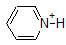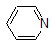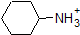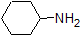# 1.14: Distinguishing between pH and pKa

Learning Objective

• Determine relative strengths of acids and bases from their pKa values
• Determine the form of an acid or base at a specified pH (given the pKa)

The Henderson-Hasselbach Equation - a Quantitative View

We will use the general reaction for a weak acid to write the Ka expression.

$HA_{(aq)} + H_2O_{(l)} \rightleftharpoons H_3O^+_{(aq)} + A^-_{(aq)}$

$K_a = \dfrac{[H_3O^+][A^-]}{[HA]}$

pKa = -log Ka

where each bracketed term represents the concentration of that substance in solution.

The stronger an acid, the greater the ionization, the lower the pKa, and the lower the pH the compound will produce in solution.

It is important to realize that pKa is not at all the same thing as pH: the former is an inherent property of a compound or functional group, while the latter is the measure of the hydronium ion concentration in a particular aqueous solution:

pH = -log [H3O+]

Additional reagents can be added to a reaction solution to change the pH of the reaction conditions beyond the effects of an individual compound.

## Relative Acidity and pKa Values

An application of the Henderson-Hasselbach Equation is the ability to determine the relative acidity of compounds by comparing their pKa values. The stronger an acid, the greater the ionization, the lower the pKa, and the lower the pH the compound will produce in solution. Some selected pKa values for compounds in the study of organic chemistry are shown bellow. Since organic reactions can be performed in non-aqueous environments, the pH can exceed 14 and organic compounds can have pKa values above 16. It is a variation on that line from the Wizard of Oz, "We don't live in water anymore."It is a very good idea to commit to memory the approximate pKa ranges of the compounds above. A word of caution: when using the pKa table, be absolutely sure that you are considering the correct conjugate acid/base pair. If you are asked to say something about the basicity of ammonia (NH3) compared to that of ethoxide ion (CH3CH2O-), for example, the relevant pKa values to consider are 9.2 (the pKa of ammonium ion) and 16 (the pKa of ethanol). From these numbers, you know that ethoxide is the stronger base. Do not make the mistake of using the pKa value of 38: this is the pKa of ammonia acting as an acid, and tells you how basic the NH2- ion is (very basic!)

* A note on the pKa of water: The pKa of water is 14. Biochemistry and organic chemistry texts often list the value as 15.7. These texts have incorrectly factored the molar value for the concentration of water into the equilibrium constant. The correct derivation of the equilibrium constant involves the activity of water, which has a value of 1.

Example

While this course begins with single functional groups, we will eventually work with interesting compounds containing multiple functional groups. Recognizing which hydrogens can be ionized as acidic protons and which hydrogens can NOT, is a useful skill. Notice in this example that we need to evaluate the potential acidity at four different locations on the molecule.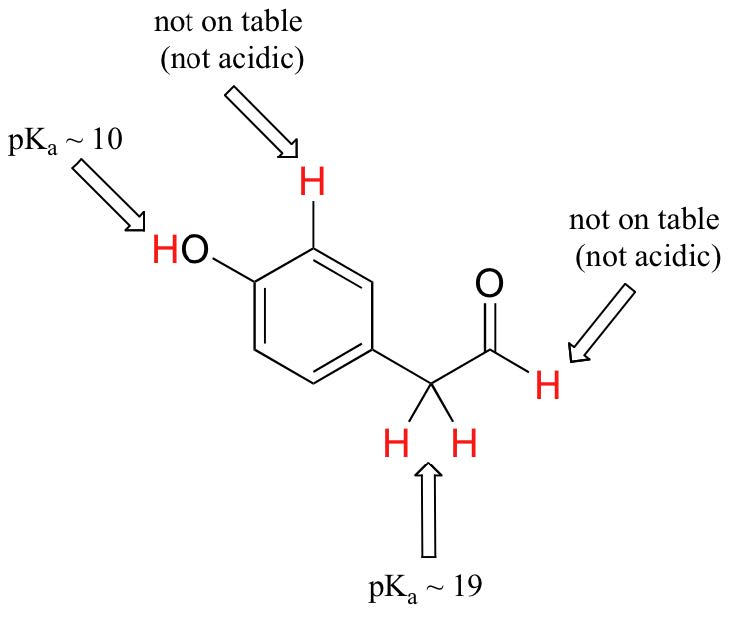Aldehyde and aromatic protons are not at all acidic (pKavalues are above 40 – not on our table). The two protons on the carbon next to the carbonyl are slightly acidic, with pKa values around 19-20 according to the table. The most acidic proton is on the phenol group, so if the compound were to be subjected to a single molar equivalent of strong base, this is the proton that would be donated.

Acidic & Basic Environments - Everything is Relative in Reactivity

Because our goal is understanding dynamic chemical reactivity, we do NOT need to know the specific amount of the protonated and unprotonated forms of a compound. We simply need to know which form is predominate. When the pH of the environment is less than the pKa of the compound, the environment is considered acidic and the compound will exist predominately in its protonated form. When the pH of the environment is greater than the pKa of the compound, the environment is considered basic and the compound will exist predominately in its deprotonated form.

For example, the pKa of acetic acid is about 5. At a pH of 1, the environment is considered acidic and acetic acid exists predominately in its protonated form. At pH 8, the environment is considered basic, and acetic acid becomes deprotonated to form acetate (CH3CO2-). Conversely, the pKa of phenol is 10. At pH 8, the environment is considered acidic for phenol and it remains primarily protonated.It is also important to remember that organic chemistry does NOT have to occur in water so pKa values can be as high as 50.

Exercise

1. Complete the table below to indicate whether each compound exists predominantly in its protonated (acidic environment) or deprotonated (basic environment) form.

 compound (pKa) pH 1 environment pH 8 environment pH 13 environment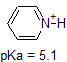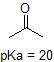compound (pKa) pH 1 environment pH 8 environment pH 13 environment